# public static int nbLeaf(BT bt, T e): This method counts and returns the number of leaf...

public static int nbLeaf(BT bt, T e):

This method counts and returns the number of leaf nodes that contain the data e. You should use the find, isLeaf and maybe otherBT methods for counting

method isLeaf

public boolean isLeaf (){
if (root==null) return false;

else

if ((current.left==null)&&(current.right==null))
return true;

else return false; }

method find :

public boolean find(Relative rel){
switch (rel) {
case Root: // Easy case
current = root;
return true;
case Parent:
if(current == root) return false;
current = current.parent;
return true;
case LeftChild:
if(current.left == null) return false;
current = current.left;
return true;
case RightChild:
if(current.right == null) return false;
current = current.right;
return true;
default:
return false;
}
}

public static int nbLeaf(BT bt, T e)

{

// if tree is empty

if( bt == null )

return 0;

// if tree is not empty

else

{

// get no of required leaf in left subtree

int l = nbLeaf( bt.left , e );

// get no of required leaf in right subtree

int r = nbLeaf( bt.right , e );

// if the current node is the required leaf node

// assuming date is the feied storing date

if( isLeaf(bt) && bt.date == e )

return l + r + 1;

// if the current node is not the required leaf node

else

return l + r;

}

}

##### Add Answer to: public static int nbLeaf(BT bt, T e): This method counts and returns the number of leaf...
Similar Homework Help Questions
• ### Using the following implementation of Tree class Node   {   public int iData;              // data item (key)   public...

Using the following implementation of Tree class Node   {   public int iData;              // data item (key)   public double dData;           // data item   public Node leftChild;         // this node's left child   public Node rightChild;        // this node's right child   public void displayNode()      // display ourself      {      System.out.print('{');      System.out.print(iData);      System.out.print(", ");      System.out.print(dData);      System.out.print("} ");      }   }  // end class Node //------------------------------------------------------------------ import java.io.IOException; import java.util.Stack; public class Tree { private Node root; // first node of tree // ------------------------------------------------------------- public Tree() // constructor { root = null; }...

• ### From the code below with Binary Search Tree recurrence T(n)=? use the recursion method and substitution method to solve the recurrence. Find the tightest bound g(n) for T(n) you can for which T(n)= O(...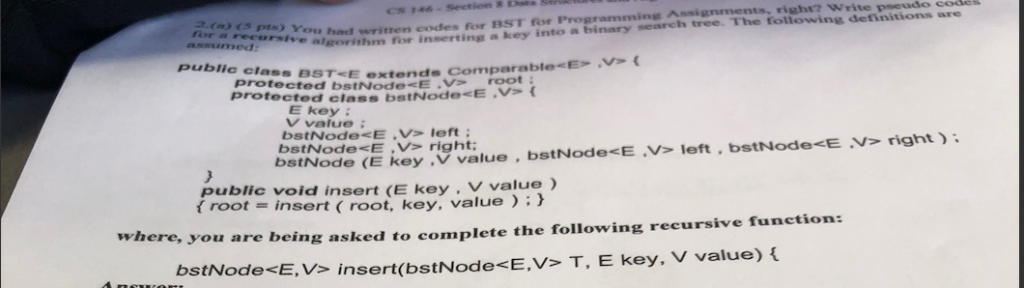From the code below with Binary Search Tree recurrence T(n)=? use the recursion method and substitution method to solve the recurrence. Find the tightest bound g(n) for T(n) you can for which T(n)= O(g(n)). Explain your answer and use recursion tree method. void insert(int data) { struct node *tempNode = (struct node*) malloc(sizeof(struct node)); struct node *current; struct node *parent; tempNode->data = data; tempNode->leftChild = NULL; tempNode->rightChild = NULL; //if tree is empty if(root == NULL) { root = tempNode;...

• ### 1) Extend the Binary Search Tree ADT to include a public method leafCount that returns the...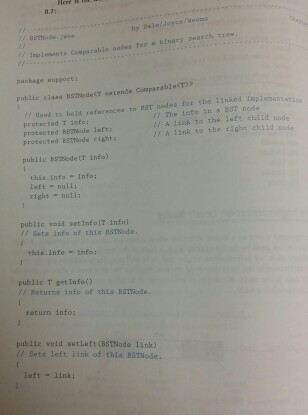1) Extend the Binary Search Tree ADT to include a public method leafCount that returns the number of leaf nodes in the tree. 2) Extend the Binary Search Tree ADT to include a public method singleParent-Count that returns the number of nodes in the tree that have only one child. 3) The Binary search tree ADT is extended to include a boolean method similarTrees that receives references to two binary trees and determines whether the shapes of the trees are...

• ### Does not pass the testcase testDTreeAdvancedReRootchangesParent Java I'm trying to extend the implementation of a general...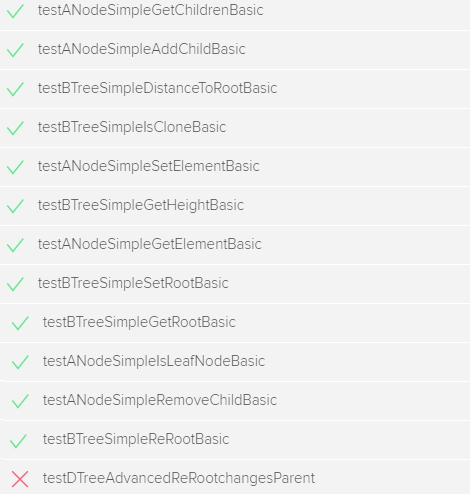Does not pass the testcase testDTreeAdvancedReRootchangesParent Java I'm trying to extend the implementation of a general tree with new operations that change the structure of the tree. I've created 5 classes: Node.java, SimpleNode.java, Tree.java, RerootableTree.java and SimpleTree.java(which is the main java class that I need to change). The code does not pass ONE TESTCASE : testDTreeAdvancedReRootchangesParent The code passes all the other testcases except theone mentioned above. This is because in the SimpleTree.java the method "reRoot(Node newRoot)" is most likely...

• ### add/ remove any methods to the program. please post new code and output Min Heap: public...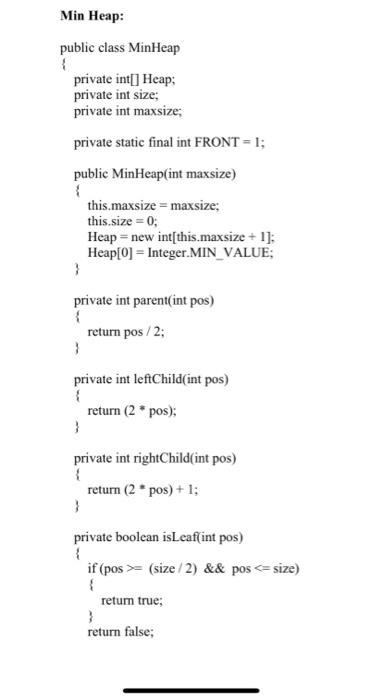add/ remove any methods to the program. please post new code and output Min Heap: public class MinHeap { private int[] Heap; private int size; private int maxsize; private static final int FRONT = 1; public MinHeap(int maxsize) { this.maxsize = maxsize; this.size = 0; Heap = new int[this.maxsize + 1]; Heap = Integer.MIN_VALUE; } private int parent(int pos) { return pos / 2; } private int leftChild(int pos) { return (2 * pos); } private int rightChild(int pos) {...

• ### Need help in the below question. Answer in java Start with the tree.java program (Listing 8.1)...

Need help in the below question. Answer in java Start with the tree.java program (Listing 8.1) and modify it to create a binary tree from a string of letters (like A, B, and so on) entered by the user. Each letter will be displayed in its own node. Construct the tree so that all the nodes that contain letters are leaves. Parent nodes can contain some non-letter symbol like +. Make sure that every parent node has exactly two children....

• ### Add a non-recursive inorder() method to class LinkedBinaryTree<E> to traverse binary tree. Test inorder() method. Implementation...

Add a non-recursive inorder() method to class LinkedBinaryTree<E> to traverse binary tree. Test inorder() method. Implementation in Java #########LinkedBinary Tree class######### public class LinkedBinaryTree<E> extends AbstractBinaryTree<E> { //---------------- nested Node class ---------------- /** Nested static class for a binary tree node. */ protected static class Node<E> implements Position<E> { private E element; // an element stored at this node private Node<E> parent; // a reference to the parent node (if any) private Node<E> left; // a reference to the left...

• ### Please I need help ASAP Java Programing: Binary Search Tree Fully implement the BST class in Listing 25.4 (on page 961 of the 11th Edition of the text). Design and write a (main) driver program to com...

Please I need help ASAP Java Programing: Binary Search Tree Fully implement the BST class in Listing 25.4 (on page 961 of the 11th Edition of the text). Design and write a (main) driver program to completely test every method in the BST class to ensure the class meets all its requirements. You should read the Listing 25.5: TestBST.java for an idea of what your program should look like. Listing 25.4 BST.java public class BST> extends AbstractTree { protected TreeNode...

• ### write a new test program called RemoveDuplicates.java. The program reads text input from keyboard or a text file and adds the words to a BST. The program then traverses the BST and prints out the word...

write a new test program called RemoveDuplicates.java. The program reads text input from keyboard or a text file and adds the words to a BST. The program then traverses the BST and prints out the words in order (based on ASCII/UNICODE order) on the screen (or to output text file). Note that you may need to make some changes to BST.java. Sample test: ----jGRASP exec: java -ea removeDuplicates Original Text: a B 2 n w C q K l 0...

• ### In Java. How would this method look? LinkedBinaryTree.java import java.util.Iterator; public class LinkedBinaryTree implements BinaryTreeADT {...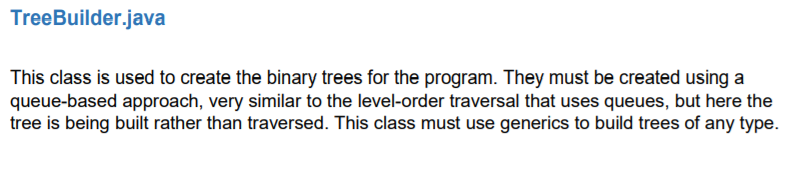In Java. How would this method look? LinkedBinaryTree.java import java.util.Iterator; public class LinkedBinaryTree implements BinaryTreeADT {    private BinaryTreeNode root;    /**    * Creates an empty binary tree.    */    public LinkedBinaryTree() {        root = null;    }    /**    * Creates a binary tree from an existing root.    */    public LinkedBinaryTree(BinaryTreeNode root) {        this.root = root;    }    /**    * Creates a binary tree with the specified element...

Free Homework App